## 5.4  Extended Phase Space

In this section we show that we can treat time as just another coordinate if we wish. Systems described by a time-dependent Hamiltonian may be recast in terms of a time-independent Hamiltonian with an extra degree of freedom. An advantage of this view is that what was a time-dependent canonical transformation can be treated as a time-independent transformation, where there are no additional conditions for adjusting the Hamiltonian.

Suppose that we have some system characterized by a time-dependent Hamiltonian, for example, a periodically driven pendulum. We may imagine that there is some extremely massive oscillator, unperturbed by the motion of the relatively massless pendulum, that produces the drive. Indeed, we may think of time itself as the coordinate of an infinitely massive particle moving uniformly and driving everything else. We often consider the rotation of the Earth as exactly such a stable time reference when performing short-time experiments in the laboratory.

More formally, consider a dynamical system with n degrees of freedom, whose behavior is described by a possibly time-dependent Lagrangian L with corresponding Hamiltonian H. We make a new dynamical system with n + 1 degrees of freedom by extending the generalized coordinates to include time and introducing a new independent variable. We also extend the generalized velocities to include a velocity for the time coordinate. In this new extended state space the coordinates are redundant, so there is a constraint relating the time coordinate to the new independent variable.

We relate the original dynamical system to the extended dynamical system as follows: Let q be a coordinate path. Let qe,t :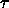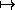qe(),t() be a coordinate path in the extended system whereis the new independent variable. Then qe = q o t, or qe() = q(t()). Consequently, if v = Dq is the velocity along a path then ve() = D qe() = Dq(t()) · Dt() = v(t()) · vt().

We can find a Lagrangian for the extended system by requiring that the value of the action be unchanged. Introduce the extended Lagrangian action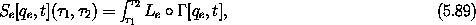with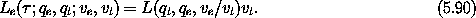We have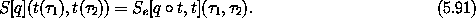The extended system is subject to a constraint that relates the time to the new independent variable. We assume the constraint is of the form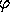(; qe, qt; ve, vt) = qt - f() = 0. The constraint is a holonomic constraint involving the coordinates and time, so we can incorporate this constraint by augmenting the Lagrangian:12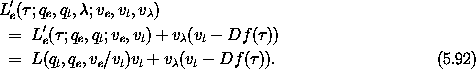The Lagrange equations of L'e for qe are satisfied for the paths q o t where q is any path that satisfies the original Lagrange equations of L.

The momenta conjugate to the coordinates are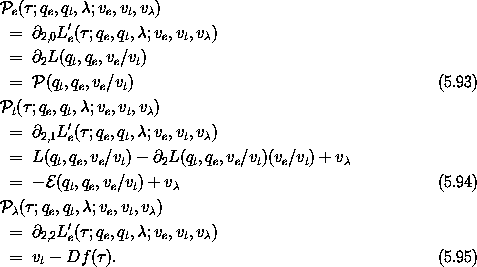So the extended momenta have the same values as the original momenta at the corresponding states. The momentum conjugate to the time coordinate is the negation of the energy plus v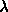. The momentum conjugate tois the constraint, which must be zero.

Next we carry out the transformation to the corresponding Hamiltonian formulation. First, note that the Lagrangian Le is a homogeneous form of degree one in the velocities. Thus, by Euler's theorem,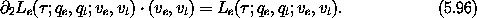The p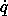-part of the Legendre transform of Le' is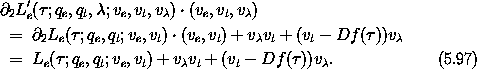So the Hamiltonian H'e corresponding to L'e is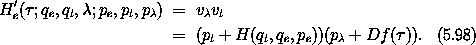We have used the fact that that at corresponding states the momenta have the same values, so on paths pe = p o t, and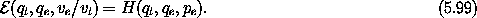The Hamiltonian H'e does not depend onso we deduce that pis constant. In fact, pmust be given the value zero, because it is the constraint. When there is a cyclic coordinate we can form a reduced Hamiltonian for the remaining degrees of freedom by substituting the constant value of conserved momentum conjugate to the cyclic coordinate into the Hamiltonian. The resulting Hamiltonian isThis extended Hamiltonian governs the evolution of the extended system, for arbitrary f.13

Hamilton's equations reduce to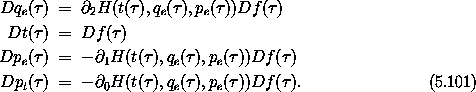The second equation gives the required relation between t and. The first and third equations are equivalent to Hamilton's equations in the original coordinates, as we can see by using qe = q o t to rewrite them: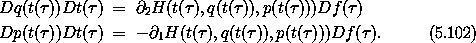Using Dt() = Df() and dividing these factors out, we recover Hamilton's equations.14

Now consider the special case for which the time is the same as the independent variable: f() =, Df() = 1. In this case q = qe and p = pe. The extended Hamiltonian becomes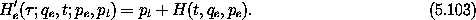Hamilton's equation for t becomes Dt() = 1, restating the constraint. The Hamilton's equations for Dqe and Dpe are directly Hamilton's equations: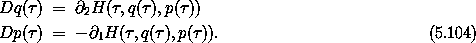The extended Hamiltonian (5.103) does not depend on the independent variable, so it is a conserved quantity. Thus, up to an additive constant pt is equal to minus the energy. The Hamilton's equation for Dpt relates the change of the energy to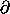0 H. Note that in the more general case, the momentum conjugate to the time is not the negation of the energy. This choice, t() =, is useful for a number of applications.

Note that the extension transformation is canonical in the sense that the two sets of equations of motion describe equivalent dynamics. However, the transformation is not symplectic; in fact, it does not even have the same number of input and output variables.

Exercise 5.10.  Homogeneous extended Lagrangian
Verify that Le is homogeneous of degree one in the velocities.

Exercise 5.11.  Lagrange equations

a.  Verify the claim that the Lagrange equations for qe are satisfied for exactly the same trajectories that satisfy the original Lagrange equations for q.

b.  Verify the claim that the Lagrange equation for t relates the rate of change of energy to0 L.

Exercise 5.12.  Lorentz transformations
Investigate Lorentz transformations as point transformations in the extended phase space.

#### Restricted three-body problem

An example that shows the utility of reformulating a problem in the extended phase space is the restricted three-body problem: the motion of a low-mass particle subject to the gravitational attraction of two other massive bodies that move in some fixed orbit. The problem is an idealization of the situation where a body with very small mass moves in the presence of two bodies with much larger masses. Any effects of the smaller body on the larger bodies are neglected. In the simplest version, the motion of all three bodies is assumed to be in the same plane, and the orbit of the two massive bodies is circular.

The motion of the bodies with larger masses is not influenced by the small mass, so we model this situation as the small body moving in a time-varying field of the larger bodies undergoing a prescribed motion. This situation can be captured as a time-dependent Hamiltonian: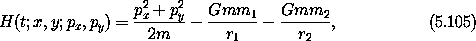where r1 and r2 are the distances of the small body to the larger bodies, m is the mass of the small body, and m1 and m2 are the masses of the larger bodies. Note that r1 and r2 are quantities that depend both on the position of the small particle and the time varying position of the massive particles.

The massive bodies are in circular orbits and maintain constant distance from the center of mass. Let a1 and a2 be the distances to the center of mass; then the distances satisfy m1 a1 = m2 a2. The angular frequency is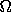= (G(m1 + m2)/a3)1/2 where a is the distance between the masses.

In polar coordinates, with the center of mass of the subsystem of massive particles at the origin and with r and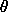describing the position of the low-mass particle, the positions of the two massive bodies are a2 = m1 a / (m1 + m2) with2 =t , a1 = m2 a / (m1 + m2) with1 =t +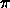. The distances to the point masses are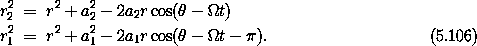So, in polar coordinates, the Hamiltonian is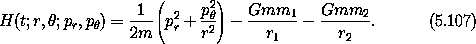We see therefore that the Hamiltonian can be written in terms of some function f such that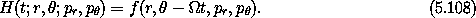The essential feature is thatand t appear in the Hamiltonian only in the combination-t.

One way to get rid of the time dependence is to choose a new set of variables with one coordinate equal to this combination-t, by making a point transformation to a rotating coordinate system. We have shown that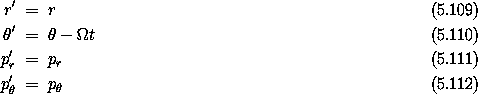with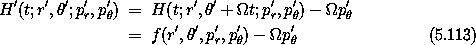is a canonical transformation. The new Hamiltonian, which is not the energy, is conserved because there is no explicit time dependence. It is a useful integral of motion -- the Jacobi constant.15

We can also eliminate the dependence on the independent time-like variable from the Hamiltonian for the restricted problem by going to the extended phase space, choosing t =. The Hamiltonian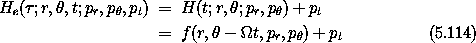is autonomous and is consequently an integral of the motion. Again, we see thatand t occur only in the combination-t, which suggests a point transformation to a new coordinate' =-t. This point transformation is independent of the new independent variable. The transformation is specified in equations (5.109-5.112), augmented by relations specifying how the time coordinate and its conjugate momentum are handled: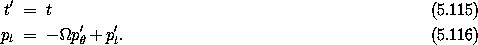The new Hamiltonian is obtained by composing the old Hamiltonian with the transformation: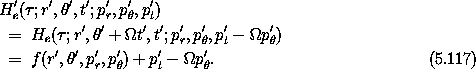We recognize that the new Hamiltonian in the extended phase space, which has the same value as the original Hamiltonian in the extended phase space, is just the Jacobi constant plus p't. The new Hamiltonian does not depend on t', so p't is a constant of the motion. In fact, its value is irrelevant to the rest of the dynamical evolution, so we may set the value of p't to zero if we like. Thus, we have found that the Hamiltonian in the extended phase space, which is conserved, is just the Jacobi constant plus an additive arbitrary constant. We have two routes to the Jacobi constant: (1) transform the original system to a rotating coordinate system to eliminate the time dependence, but in the process add extra terms to the Hamiltonian, and (2) go to the extended phase space and immediately get an integral, and by going to rotating coordinates recognize that this Hamiltonian is the same as the Jacobi constant. So sometimes the Hamiltonian in the extended phase space is a useful integral.

Exercise 5.13.  Transformations in the extended phase space
In section 5.2.3 we found that time-dependent transformations for which the derivative of the coordinate-momentum part is symplectic are canonical only if the Hamiltonian is modified by adding a function K subject to certain constraints (equation 5.54). Show that the constraints on K follow from the symplectic condition in the extended phase space, using the choice t =.

### 5.4.1  Poincaré-Cartan Integral Invariant

A time-dependent transformation is canonical if in the extended phase space the Hamiltonians transform by composition and the extended phase-space transformation is symplectic. In section 5.3 we showed that if the derivative of the transformation is symplectic then the sum of the areas of the projections of any two-dimensional region of phase space onto the canonical qi, pi planes is preserved. This invariance is also true of symplectic transformations in the extended phase space. Let R and R' be corresponding regions of extended phase-space coordinates. Let Ai be the area of the projection of the region R onto the canonical qi, pi plane, and A'i be the area of the projection of the corresponding region R' onto the canonical q'i, p'i plane. In the extended phase space, we also have a projection onto the t, pt canonical plane. Let An be the area of the projection onto the t, pt plane. We have then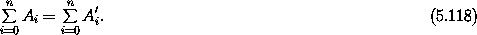In terms of integrals this is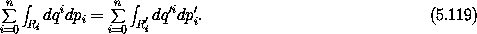This equality for the sum of area integrals can be rewritten in terms of line integrals by Stokes's theorem: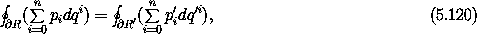where the order of the integration and summation can be reversed because the boundary of R projects to the boundary on the canonical planes.

For the special choice of t =this result can be rephrased in an interesting way. Let E be the value of the Hamiltonian in the original unextended phase space. Using qn = t and pn = pt = - E, we can write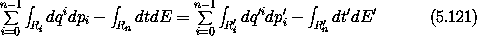and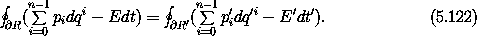The relations (5.121) and (5.122) are two formulations of the Poincaré-Cartan integral invariant.

12 We augment the Lagrangian with the total time derivative of the constraint so that the Legendre transform will be well defined.

13 Once we have made this reduction, taking pto be zero, we can no longer perform a Legendre transform back to the extended Lagrangian system; we cannot solve for pt in terms of vt. However, the Legendre transform in the extended system from H'e to L'e, with associated state variables, is well defined.

14 If f is strictly increasing then Df is never zero.

15 Actually, the traditional Jacobi constant is C = - 2 H'.